• 计算机视觉的实验，Python写的代码，萌新代码，勿喷，仅仅只是方便没时间写实验的朋友，直接用python打开就能运行
• ''' .... # 点可代表一切字符 \ # 起转义作用 [...] # 指代方括号中的任意字符 \d # 指代数字0-9 ...\s # 指代一切空格，包括tab制表符、空格、换行等...* # 匹配前面字符 >=0 次 + # 匹配前面字符1次及以上 ? # 匹..
'''
https://www.cnblogs.com/syw20170419/p/9749809.html
. # 点可代表一切字符

\ # 起转义作用

[...] # 指代方括号中的任意字符

\d # 指代数字0-9

\D # 指代非数字

\s # 指代一切空格，包括tab制表符、空格、换行等

\S # 指代非空格

\w # 指代大小写字母、数字和下划线

\W # 指代非大小写字母、数字和下划线

* # 匹配前面字符 >=0 次

+ # 匹配前面字符1次及以上

? # 匹配前面字符0次或1次

{m} # 匹配m次

{m,n} # 匹配m到n次

{m,} # 至少匹配m次
'''

import re

my_str = "abc .abc .abc1-abc"
old="abc"
new = "xxx"

result = re.sub("\\b" + old + "\\b", new, my_str)
result = re.sub(r"\b%s\b" % (old), new, my_str)
result = re.sub("\." + "\\b" + "abc" + "\\b", new, my_str)
result1 = re.findall("\." + "\\b" + "abc" + "\\b",my_str)
print(result1)
展开全文正则表达式 深度学习
• SAD进行双目立体视觉匹配Python语言）1 编译环境2 算法原理介绍3 基本流程3.1 步骤3.2 示意图4 代码（Python）5 运行结果6 结论 1 编译环境 编程语言：Python IDE：PyCharm 2017 2 算法原理介绍 SAD(Sum of ...


1 编译环境2 算法原理介绍3 基本流程3.1 步骤3.2 示意图
4 代码（Python）5 运行结果6 结论

1 编译环境
编程语言：Python IDE：PyCharm 2017
2 算法原理介绍
3 基本流程
3.1 步骤
输入：两幅图像，一般为左相机和右相机得到得左图和右图，分别设置为Left-Image，Right-Image 遍历左图中得所有像素点，并对每一个像素点执行如下操作： （1）构造一个小窗口,类似于卷积核； （2）用窗口覆盖左边的图像,选择出窗口覆盖区域内的所有像素点； （3）同样用窗口覆盖右边的图像并选择出覆盖区域的像素点； （4）左边覆盖区域减去右边覆盖区域，并求出所有像素点灰度差的绝对值之和； （5）移动右边图像的窗口，重复（3）-（4）的处理（这里有个搜索范围,超过这个范围跳出）； （6）找到这个范围内SAD值最小的窗口,即找到了左图锚点的最佳匹配的像素块。
3.2 示意图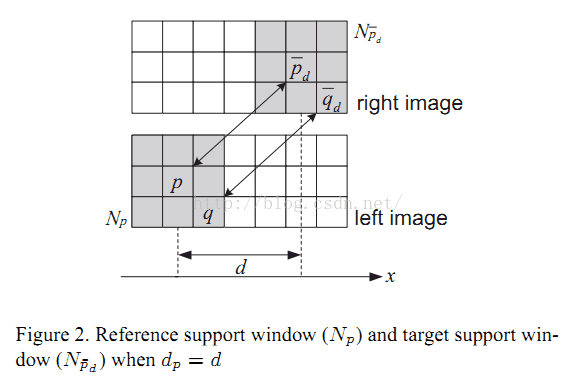4 代码（Python）
import cv2
from pylab import *
import time

width,height=Img_L.shape
kernel_L=np.zeros((winsize,winsize),dtype='uint8')
kernel_R = np.zeros((winsize, winsize), dtype='uint8')
disparity=np.zeros((width, height), dtype='uint8')
for i in range(width-winsize):
for j in range(height-winsize):
kernel_L=Img_L[i:i+winsize,j:j+winsize]
v=*DSR
for k in range(DSR):
x=i-k
if x>=0:
kernel_R=Img_R[x:x+winsize,j:j+winsize]
for m in range(winsize):
for n in range(winsize):
v[k]=v[k]+abs(kernel_R[m,n]-kernel_L[m,n])
disparity[i,j]=min(v)
return disparity
start=time.process_time()                   #获取代码运行时间
cv2.imshow('Origion_L',img_L)
cv2.imshow('Origion_R',img_R)
cv2.waitKey()
cv2.destroyAllWindows()
end=time.process_time()
print('Running time:',end-start)            #显示运行时间
5 运行结果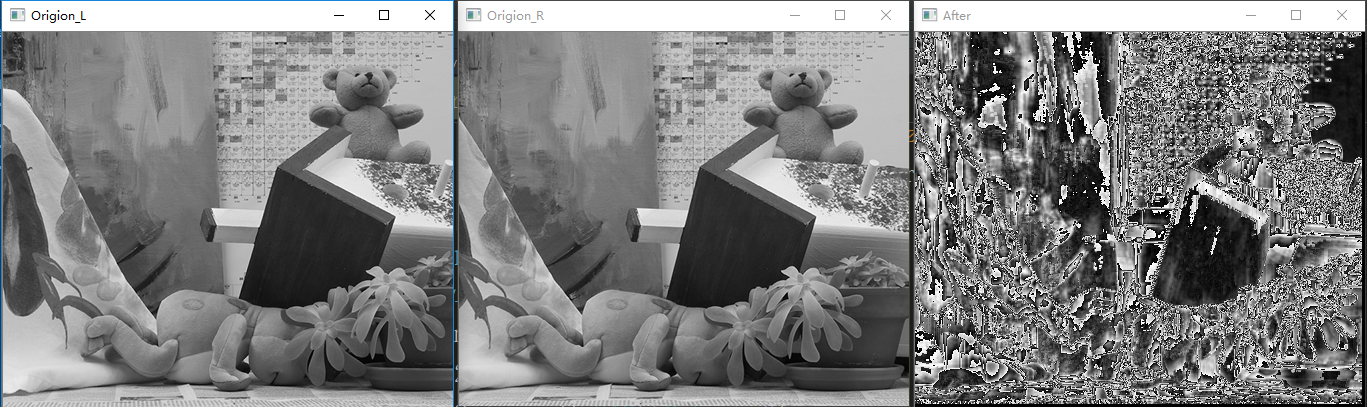6 结论
处理效果不好得原因应该是与卷积核得大小有关，可设置对照实验验证之。 csdn中第一篇python语言编写的SAD双目立体匹配文章，多谢阅读，可评论区交流。
展开全文数字图像处理
• 使用python3.7实现SGBM算法，通过调用opencv库函数来实现图像匹配，计算出左右图的视差图
• 立体匹配1.1 概述1.2 主要立体匹配算法分类1.3 立体匹配的基本步骤2. 归一化互相关（NCC）视差匹配法2.1 原理2.2 匹配流程：3. 不同窗口值对匹配结果的影响 实验要求： 从理论角度，分析以窗口代价计算视差的原理 ...


文章目录
1. 立体匹配1.1 概述1.2 主要立体匹配算法分类1.3 立体匹配的基本步骤
2. 归一化互相关（NCC）视差匹配法2.1 原理2.2 匹配流程2.3 代码实现
3. 不同窗口值对匹配结果的影响4. 实验遇到的问题与解决

1. 立体匹配
1.1 概述
立体匹配是立体视觉研究中的关键部分。其目标是在两个或多个视点中匹配相应像素点，计算视差。通过建立一个能量代价函数，对其最小化来估计像素点的视差，求得深度。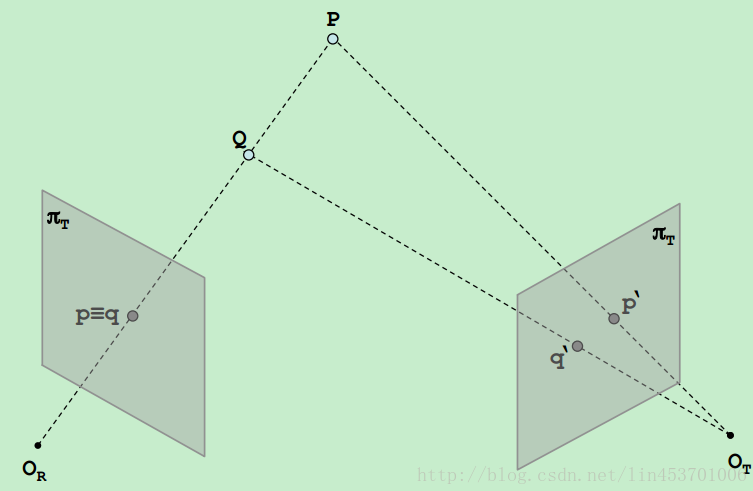点P和Q，映射到左相机OR像面上的同一点p≡q，只要找到p和q在右相机OT像面上的对应点就可以通过三角计算估计深度。找到对应点的过程，即立体匹配。
为了找到对应点，需要增加约束，最常用的是极线约束。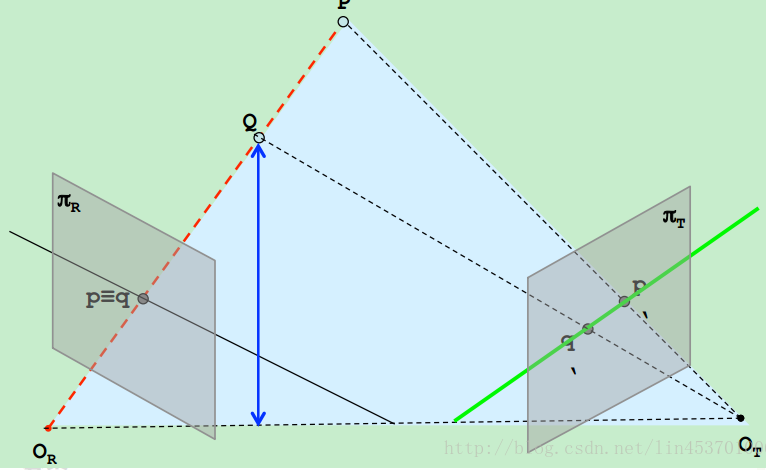P和Q映射到左相机QR像面上的同一点p≡q，直线pq上的点对应点一定位于右像面的直线p’q’上，p’q’即为直线pq的极线，这就是极线约束。
接下来就可以根据视差估计深度，然后通过Graph cuts算法给每一个像素点分配视差从而得到深度图，不再详细说明。
1.2 主要立体匹配算法分类
1）根据采用图像表示的基元不同，立体匹配算法分为： A、区域立体匹配算法：可获取稠密视差图。缺点：受图像的仿射畸变和辐射畸变影响较大；像素点约束窗口的大小与形状选择比较困难，选择过大，在深度不连续处，视差图中会出现过度平滑现象；选择过小，对像素点的约束比较少，图像信息没有得到充分利用，容易产生误匹配。 B、基于特征的立体匹配算法：可获得稀疏的视差图，经差值估计可获得稠密视差图。可提取点、线、面等局部特征，也可提取多边形和图像结构等全局特征。缺点：特征提取易受遮挡、光线、重复纹理等影响较大；差值估计计算量大。 C、基于相位立体匹配算法：假定在图像对应点中，其频率范围内，其局部相位是相等的，在频率范围内进行视差估计。 2）依据采用最优化理论方法的不同，立体匹配算法可以分为： A、局部的立体匹配算法 B、全局的立体匹配算法
还有立体匹配算法介绍的更详细内容：参考博客
1.3 立体匹配的基本步骤
立体匹配过程：
2. 归一化互相关（NCC）视差匹配法
2.1 原理
对于原始的图像内任意一个像素点

(

p

x

,

p

y

)

(px,py)

构建一个

n

×

n

n×n

的邻域作为匹配窗口。然后对于目标相素位置

(

p

x

+

d

,

p

y

)

(px+d,py)

同样构建一个

n

×

n

n×n

大小的匹配窗口，对两个窗口进行相似度度量，注意这里的dd dd有一个取值范围。对于两幅图像来说，在进行

N

C

C

NCC

计算之前要对图像处理，也就是将两帧图像校正到水平位置，即光心处于同一水平线上，此时极线是水平的，否则匹配过程只能在倾斜的极线方向上完成，这将消耗更多的计算资源。

N

C

C

NCC

计算公式如下：

N

C

C

(

p

,

d

)

=

∑

(

x

,

y

)

∈

W

p

(

I

1

(

x

,

y

)

−

I

1

‾

(

P

x

,

P

y

)

)

⋅

(

I

1

(

x

+

d

,

y

)

−

I

2

‾

(

P

x

+

d

,

P

y

)

)

∑

(

x

,

y

)

∈

W

p

(

I

1

(

x

,

y

)

−

I

1

‾

(

P

x

,

P

y

)

)

2

⋅

∑

(

x

,

y

)

∈

W

p

(

I

2

(

x

+

d

,

y

)

−

I

2

‾

(

P

x

+

d

,

P

y

)

)

2

NCC(p,d)=\frac{\sum_{(x,y)\in W_{p}}(I_{1}(x,y)-\overline{I_{1}}(P_{x},P_{y}))\cdot (I_{1}(x+d,y)-\overline{I_{2}}(P_{x}+d,P_{y}))}{\sum_{(x,y)\in W_{p}}(I_{1}(x,y)-\overline{I_{1}}(P_{x},P_{y}))^2\cdot \sum_{(x,y)\in W_{p}}(I_{2}(x+d,y)-\overline{I_{2}}(P_{x}+d,P_{y}))^2}

其中

N

C

C

(

p

,

d

)

NCC(p,d)

得到的值的范围将在[−1,1]之间。

W

p

Wp

为之前提到的匹配窗口。

I

1

(

x

,

y

)

I_{1}(x,y)

为原始图像的像素值。

I

1

‾

(

p

x

,

p

y

)

\overline{I_{1}}(p_x,p_y)

为原始窗口内像素的均值。

I

2

(

x

+

d

,

y

)

I_{2}(x+d,y)

为原始图像在目标图像上对应点位置在

x

x

方向上偏移

d

d

后的像素值。

I

2

‾

(

p

x

+

d

,

p

y

)

\overline{I_{2}}(p_x+d, p_y)

为目标图像匹配窗口像素均值。
若

N

C

C

=

−

1

NCC=−1

，则表示两个匹配窗口完全不相关，相反，若

N

C

C

=

1

NCC=1

时，表示两个匹配窗口相关程度非常高。
2.2 匹配流程
采集图像：通过标定好的双目相机采集图像，当然也可以用两个单目相机来组合成双目相机。  极线校正：校正的目的是使两帧图像极线处于水平方向，或者说是使两帧图像的光心处于同一水平线上。通过校正极线可以方便后续的

N

C

C

NCC

操作。 1）由标定得到的内参中畸变信息中可以对图像去除畸变。 2）通过校正函数校正以后得到相机的矫正变换R和新的投影矩阵P，接下来是要对左右视图进行去畸变，并得到重映射矩阵。  特征匹配：这里便是我们利用

N

C

C

NCC

做匹配的步骤啦，匹配方法如上所述，右视图中与左视图待测像素同一水平线上相关性最高的即为最优匹配。完成匹配后，我们需要记录其视差

d

d

，即待测像素水平方向

x

l

xl

与匹配像素水平方向

x

r

xr

之间的差值

d

=

x

r

−

x

l

d=xr−xl

，最终我们可以得到一个与原始图像尺寸相同的视差图

D

D

深度恢复：通过上述匹配结果得到的视差图

D

D

，我们可以很简单的利用相似三角形反推出以左视图为参考系的深度图。计算原理如下图所示：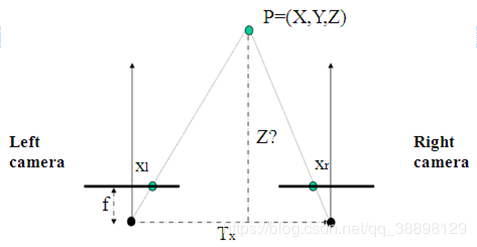如图，

T

x

Tx

为双目相机基线，

f

f

为相机焦距，这些可以通过相机标定步骤得到。而

x

r

−

x

l

xr−xl

就是视差

d

d

。 通过公式

z

=

f

×

T

x

d

z=\frac{f×Tx}{d}

可以很简单地得到以左视图为参考系的深度图了。 至此，我们便完成了双目立体匹配。倘若只是用于图像识别，那么到步骤3时已经可以结束了。
2.3 代码实现
NCCfaster.py
import numpy as np
import cv2
from PCV.localdescriptors import sift

im1 = 'C://Users//Garfield//PycharmProjects//untitled//NCC-master//im2.ppm'
im2 = 'C://Users//Garfield//PycharmProjects//untitled//NCC-master//im6.ppm'

rows, cols = img1.shape
print(img1.shape)

def translaton(image, shape):
step = round((shape-1)/2)
print(step)
shifted = []
for i in range(0, step+1):
for j in range(0, step+1):
if i==0 and j==0:
M1 = np.float32([[1, 0, i], [0, 1, j]])
shifted.append(cv2.warpAffine(image, M1, (image.shape, image.shape)))
elif i==0 and j!=0:
M1 = np.float32([[1, 0, i], [0, 1, j]])
M2 = np.float32([[1, 0, i], [0, 1, -j]])
shifted.append(cv2.warpAffine(image, M1, (image.shape, image.shape)))
shifted.append(cv2.warpAffine(image, M2, (image.shape, image.shape)))
elif i!=0 and j==0:
M1 = np.float32([[1, 0, i], [0, 1, j]])
M2 = np.float32([[1, 0, -i], [0, 1, j]])
shifted.append(cv2.warpAffine(image, M1, (image.shape, image.shape)))
shifted.append(cv2.warpAffine(image, M2, (image.shape, image.shape)))
else:
M1 = np.float32([[1, 0, i], [0, 1, j]])
M2 = np.float32([[1, 0, -i], [0, 1, -j]])
M3 = np.float32([[1, 0, -i], [0, 1, j]])
M4 = np.float32([[1, 0, i], [0, 1, -j]])
shifted .append(cv2.warpAffine(image, M1, (image.shape, image.shape)))
shifted.append(cv2.warpAffine(image, M2, (image.shape, image.shape)))
shifted.append(cv2.warpAffine(image, M3, (image.shape, image.shape)))
shifted.append(cv2.warpAffine(image, M4, (image.shape, image.shape)))

print(len(shifted))
return np.array(shifted)

#I(x,y)-avg(I(x,y))
def img_sub_avg(img_shifted, avg_img):
len, height, width = img1_shifted.shape
tmp_ncc1 = np.zeros([len, height, width])
for i in range(len):
tmp_ncc1[i] = img_shifted[i] - avg_img
print(tmp_ncc1)
return tmp_ncc1

def NCC(img1_sub_avg,img2_sub_avg, threshold, max_d):
#设立阈值
len, height, width = img1_sub_avg.shape
thershould_shifted = np.zeros([len, height, width])
ncc_max = np.zeros([height, width])
ncc_d = np.zeros([height, width])
for j in range(3, max_d):
tmp_ncc1 = np.zeros([height, width])
tmp_ncc2 = np.zeros([height, width])
tmp_ncc3 = np.zeros([height, width])
for k in range(len):
M1 = np.float32([[1, 0, -j - 1], [0, 1, 0]])
thershould_shifted[k] = cv2.warpAffine(img1_sub_avg[k], M1, (img1_sub_avg.shape, img1_sub_avg.shape))
for i in range(len):
tmp_ncc1 += (img2_sub_avg[i])*(thershould_shifted[i])
tmp_ncc2 += pow(img2_sub_avg[i], 2)
tmp_ncc3 += pow(thershould_shifted[i], 2)

tmp_ncc2 = tmp_ncc2*tmp_ncc3
tmp_ncc2 = np.sqrt(tmp_ncc2)
tmp_ncc4 = tmp_ncc1/tmp_ncc2
for m in range(height):
for n in range(width):
if tmp_ncc4[m, n] > ncc_max[m ,n] and tmp_ncc4[m, n] > threshold:
ncc_max[m, n] = tmp_ncc4[m, n]
ncc_d[m , n] = j
for i in ncc_d:
print(i)
return ncc_max, ncc_d

if __name__ == "__main__":
disparity = np.zeros([rows, cols])
NCC_value = np.zeros([rows, cols])
deeps = np.zeros([rows, cols])
# 用3*3卷积核做均值滤波
avg_img1 = cv2.blur(img1, (7, 7))
avg_img2 = cv2.blur(img2, (7, 7))
fimg1 = img1.astype(np.float32)
fimg2 = img2.astype(np.float32)
avg_img1 = avg_img1.astype(np.float32)
avg_img2  = avg_img2.astype(np.float32)
img1_shifted = translaton(fimg1, [7, 7])
img2_shifted = translaton(fimg2, [7, 7])
img1_sub_avg = img_sub_avg(img1_shifted, avg_img1)
img2_sub_avg = img_sub_avg(img2_shifted, avg_img2)
ncc_max, ncc_d = NCC(img1_sub_avg,img2_sub_avg, threshold = 0.5, max_d = 64)
print(img1_shifted.shape)
disp = cv2.normalize(ncc_d, ncc_d, alpha=0, beta=255, norm_type=cv2.NORM_MINMAX,
dtype=cv2.CV_8U)
cv2.imshow("left", img1)
cv2.imshow("right", img2)
cv2.imshow("depth", disp)
cv2.waitKey(0)  # 等待按键按下
cv2.destroyAllWindows()#清除所有窗口

原始图像：运行结果：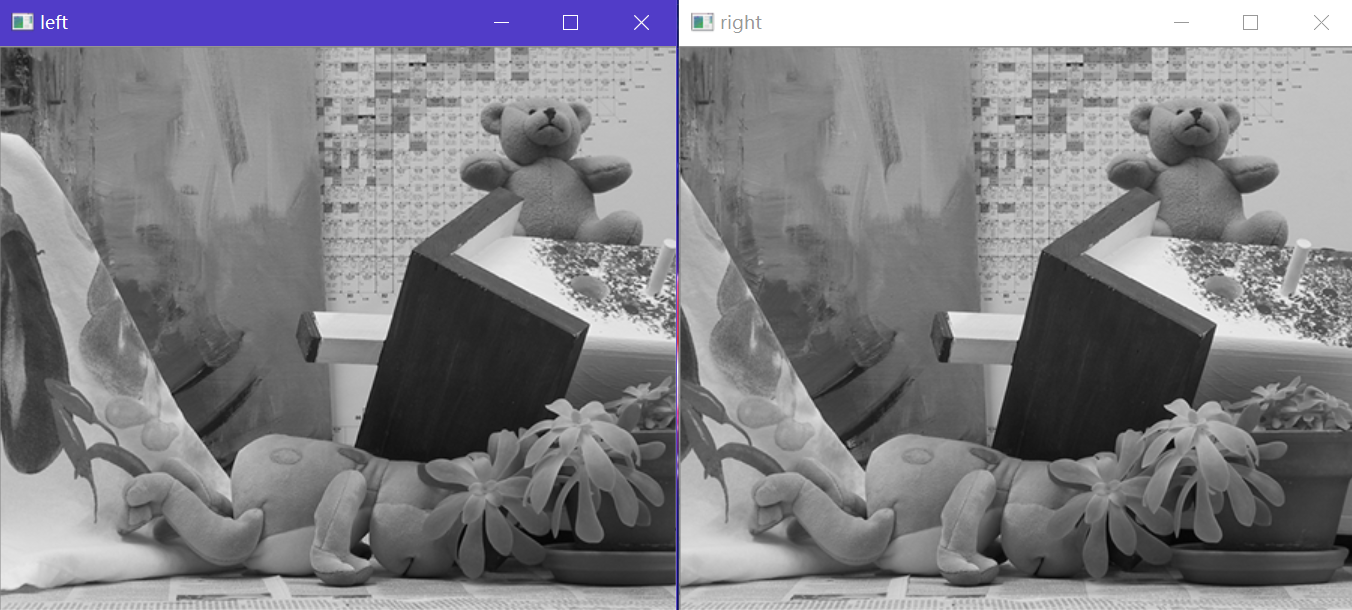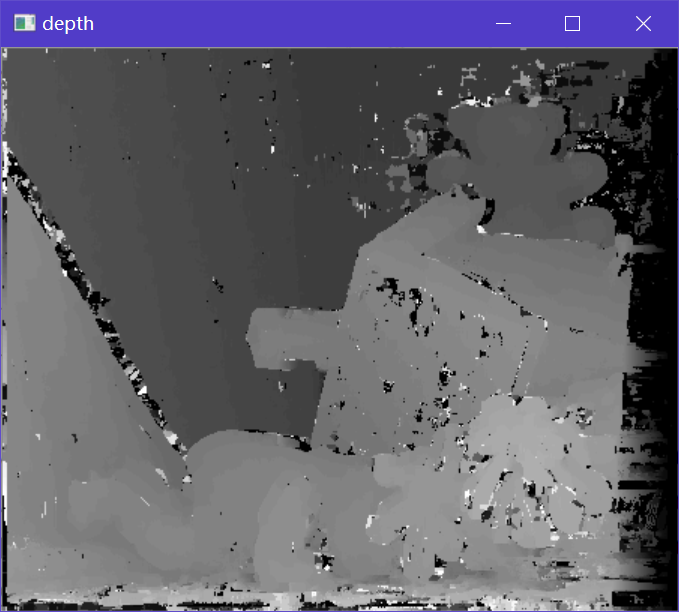代码2：
# -*- coding: utf-8 -*-
import scipy.misc
from PIL import Image
from pylab import *
import cv2
from numpy import *
from numpy.ma import array
from scipy.ndimage import filters
def plane_sweep_ncc(im_l,im_r,start,steps,wid):
""" 使用归一化的互相关计算视差图像 """
m,n = im_l.shape
# 保存不同求和值的数组
mean_l = zeros((m,n))
mean_r = zeros((m,n))
s = zeros((m,n))
s_l = zeros((m,n))
s_r = zeros((m,n))
# 保存深度平面的数组
dmaps = zeros((m,n,steps))
# 计算图像块的平均值
filters.uniform_filter(im_l,wid,mean_l)
filters.uniform_filter(im_r,wid,mean_r)
# 归一化图像
norm_l = im_l - mean_l
norm_r = im_r - mean_r
# 尝试不同的视差
for displ in range(steps):
# 将左边图像移动到右边，计算加和
filters.uniform_filter(np.roll(norm_l, -displ - start) * norm_r, wid, s) # 和归一化
filters.uniform_filter(np.roll(norm_l, -displ - start) * np.roll(norm_l, -displ - start), wid, s_l)
filters.uniform_filter(norm_r*norm_r,wid,s_r) # 和反归一化
# 保存 ncc 的分数
dmaps[:,:,displ] = s / sqrt(s_l * s_r)
# 为每个像素选取最佳深度
return np.argmax(dmaps, axis=2)

def plane_sweep_gauss(im_l,im_r,start,steps,wid):
""" 使用带有高斯加权周边的归一化互相关计算视差图像 """
m,n = im_l.shape
# 保存不同加和的数组
mean_l = zeros((m,n))
mean_r = zeros((m,n))
s = zeros((m,n))
s_l = zeros((m,n))
s_r = zeros((m,n))
# 保存深度平面的数组
dmaps = zeros((m,n,steps))
# 计算平均值
filters.gaussian_filter(im_l,wid,0,mean_l)
filters.gaussian_filter(im_r,wid,0,mean_r)
# 归一化图像
norm_l = im_l - mean_l
norm_r = im_r - mean_r
# 尝试不同的视差
for displ in range(steps):
# 将左边图像移动到右边，计算加和
filters.gaussian_filter(np.roll(norm_l, -displ - start) * norm_r, wid, 0, s) # 和归一化
filters.gaussian_filter(np.roll(norm_l, -displ - start) * np.roll(norm_l, -displ - start), wid, 0, s_l)
filters.gaussian_filter(norm_r*norm_r,wid,0,s_r) # 和反归一化
# 保存 ncc 的分数
dmaps[:,:,displ] = s / np.sqrt(s_l * s_r)
# 为每个像素选取最佳深度
return np.argmax(dmaps, axis=2)

im_l = array(Image.open(r'C://Users//Garfield//Desktop//towelmatch//jidian//1.jpg').convert('L'), 'f')
im_r = array(Image.open(r'C://Users//Garfield//Desktop//towelmatch//jidian//2.jpg').convert('L'),'f')
# 开始偏移，并设置步长
steps = 12
start = 4
# ncc 的宽度
wid = 2000
res = plane_sweep_ncc(im_l,im_r,start,steps,wid)

imsave('C://Users//Garfield//PycharmProjects//untitled//NCC-master//depth2000.png',res)
show()


此代码可以修改窗口值的大小，具体结果与分析放在下一小节
3. 不同窗口值对匹配结果的影响
原图：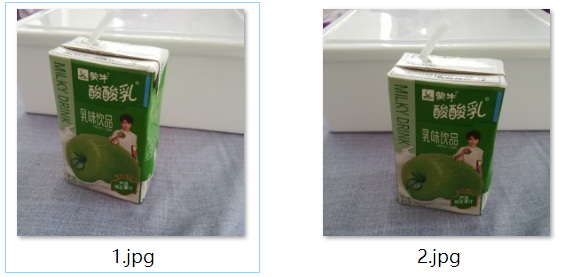以下分别是当窗口值设定为15，20，50，100，200，500，700，1000，2000的运行结果：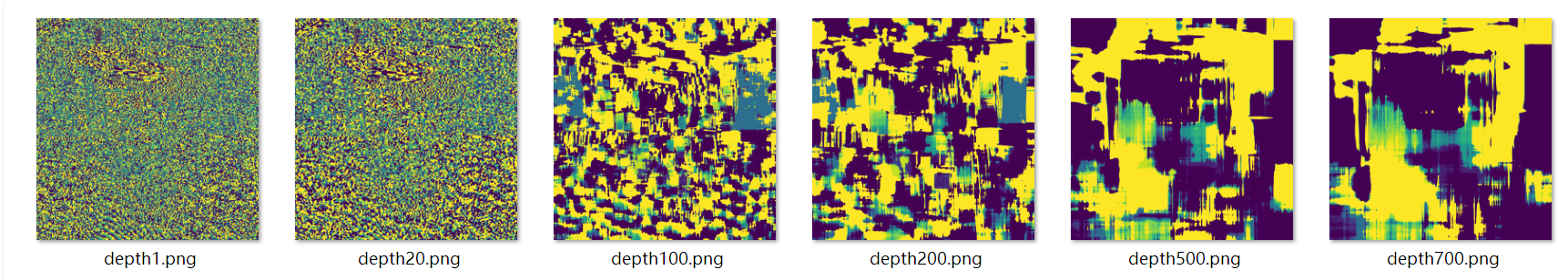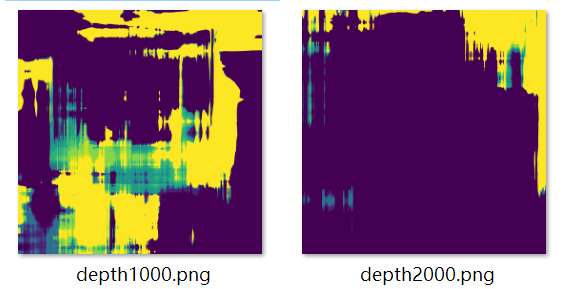总的来看，窗口值设置的越大，区域化分得越为明显，而设置的越小噪声越大，区块划分的越不明显。同时，从本次实验结果图来看，wid设置为700时，结果最为理想。 另外可以看到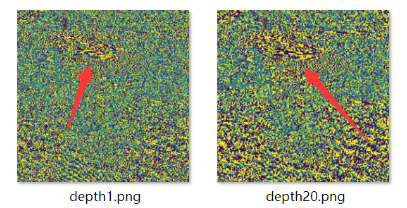瓶身光照最为强烈的地方会与瓶身其他其他地方像素值有较大区分，以及窗口大小设置越大，则会更多的提取出有光照的像素点并连结成块，区分出亮部暗部。
4. 实验遇到的问题与解决
问题：
‘NoneType’ object has no attribute ‘shape’
求解cols,rows = img.shape时出现上述报错，是图片路径不对，程序无法获取到图片。
解决办法： 右键下图目录灰色处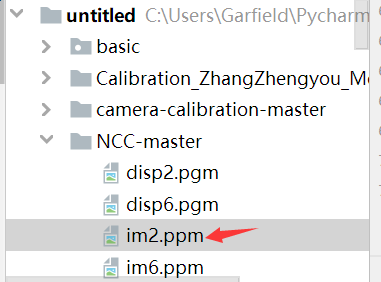—>copypath,再在原本的读取图片路径代码中粘贴 注意格式改成C://Users//… 参考博客：立体匹配_数据结构与算法 参考博客：立体匹配过程 参考博客:双目立体匹配算法–归一化互相关（NCC）详解和代码实现（python) NCC的更详细相关 延伸学习：真实场景的双目立体匹配（Stereo Matching）获取深度图详解 原理详解：双目视觉（三）立体匹配算法
展开全文• ## python 模板匹配

千次阅读 2019-07-09 23:19:18
不需要的部分，可以用黑色填充，但是返回的依然是矩形框。


import cv2

cv2.imshow("template",template)
theight, twidth = template.shape[:2]
#执行模板匹配，采用的匹配方式cv2.TM_SQDIFF_NORMED
result = cv2.matchTemplate(target,template,cv2.TM_SQDIFF_NORMED)

cv2.normalize( result, result, 0, 1, cv2.NORM_MINMAX, -1 )
#寻找矩阵（一维数组当做向量，用Mat定义）中的最大值和最小值的匹配结果及其位置
min_val, max_val, min_loc, max_loc = cv2.minMaxLoc(result)
#匹配值转换为字符串
#对于cv2.TM_SQDIFF及cv2.TM_SQDIFF_NORMED方法min_val越趋近与0匹配度越好，匹配位置取min_loc
#对于其他方法max_val越趋近于1匹配度越好，匹配位置取max_loc
strmin_val = str(min_val)
#绘制矩形边框，将匹配区域标注出来
#min_loc：矩形定点
#(min_loc+twidth,min_loc+theight)：矩形的宽高
#(0,0,225)：矩形的边框颜色；2：矩形边框宽度
cv2.rectangle(target,min_loc,(min_loc+twidth,min_loc+theight),(0,0,225),2)
#显示结果,并将匹配值显示在标题栏上
cv2.imshow("MatchResult--Value"+strmin_val,target)

cv2.waitKey()
cv2.imwrite(r"e:/new/14.jpg",target)
cv2.destroyAllWindows()

展开全文• 基于双目立体视觉的图像匹配与测距Python代码，可以运行。
• 使用模板匹配查找图像中的对象 函数：cv.matchTemplate()，cv.minMaxLoc() 理论 模板匹配是一种在较大图像中搜索和查找模板图像位置的方法。为此，OpenCV附带了一个函数cv.matchTemplate()。它只是在输入图像上滑动...opencv 正则表达式 java
• ## python 模板匹配对比

千次阅读 2019-07-10 11:39:54
平方差匹配CV_TM_SQDIFF：用两者的平方差来匹配，最好的匹配值为0 归一化平方差匹配CV_TM_SQDIFF_NORMED 相关匹配CV_TM_CCORR：用两者的乘积匹配，数值越大表明匹配程度越好 归一化相关匹配CV_TM_CCORR_NO...
• 简单来说模板匹配就是通过现有的模板去与图片进行比较找出图中所匹配的图像。 首先来看一下效果图： 左图是模板图片，中图是带测试的图片，右图是匹配的结果 程序将在“中图”中匹配“左图”的模板图片，...opencv
• 该操作不仅经常应用在计算机图形学中，而且经常出现在计算机视觉算法中。 一、仿射变换原理 仿射变换能够保持图像的“平直性”，包括旋转，缩放，平移，错切操作。对于三个点，仿射变换可以将一副图像进行扭曲，使得...
• 本文我们将使用局部描述子来匹配带有地理标记的图像： • 输入同一场景的序列图像 • 通过SIFT算法对地理标记图像进行两两匹配...1.在python中安装graphviz，用 pip install graphviz 下载。 2.可以去官网 https://...
• 可在Windows环境中使用，利用opencv和vs软件，环境配置好之后，更改程序中的相应图片，就可以得到双目视觉的视差图
• 特征检测与匹配的目标是识别一个图像中的关键点与另一个图像中的对应点之间的配对。在此实验中，你将编写代码以检测图像中的特征点（对于平移、旋转和照明具有一定的不变性），并在另一个图像中找到最佳匹配特征。...
• 平方差匹配CV_TM_SQDIFF：用两者的平方差来匹配，最好的匹配值为0 归一化平方差匹配CV_TM_SQDIFF_NORMED 相关匹配CV_TM_CCORR：用两者的乘积匹配，数值越大表明匹配程度越好 归一化相关匹配CV_TM_CCORR_NORMED ...
• 我们使用一个查询图像，在其中找到一些特征点（关键点），我们又在另一幅图像中也找到了一些特征点，最后对这两幅图像之间的特征点进行匹配。简单来说就是：我们在一张杂乱的图像中找到了一个对象（的某些部分）的...算法 java 人工智能
• ===================================================== ...==================================================...计算机视觉与深度学习 | ORB特征提取：基于OpenCV+Python（附代码） 2011年Rublee等提出了ORB（Oropencv 算法
• 我用cv2.matchtemplate无论匹配成功还是失败都会返回一个矩阵，求教如何判定匹配是成功还是失败opencv

千次阅读 热门讨论 2020-02-09 20:46:54opencv 算法
• 它轻量级而且高效——由一系列 C 函数和少量 C++ 类构成，同时提供了Python、Ruby、MATLAB等语言的接口，实现了图像处理和计算机视觉方面的很多通用算法。 今天我们就是用python学习OpenCV。...图像识别
• 这篇博文将实现如何将标准模板匹配扩展到多尺度，从而使其更加健壮。使其可以处理模板和输入图像大小不同的匹配。 我们还发现，在模板图像是刚性和格式良好的情况下，使用边缘映射而不是RGB或灰度表示可以在应用...opencv 图像处理
• 本人新书《玩转Python网络爬虫》，可在天猫、京东等商城搜索查阅，项目深入浅出，适合爬虫初学者或者是已经有一些网络爬虫编写经验，但希望更加全面、深入理解Python爬虫的开发人员。 ———-欢迎加入学习交流QQ...人工智能
• matlab匹配滤波代码Python中的视觉Odometry管道 用于移动机器人的视觉算法UZH HS 2020。 作者：EduardČuba 课程网站： 演示版 停车处： KITTI： 安装 需要Python 3.7+。 快速开始： pip3 install virtualenv ...
• 局部图像描述子Harris角点原理代码实现SIFT原理代码实现匹配地理标记图像 Harris角点 原理 代码实现 from pylab import * from PIL import Image from scipy.ndimage import filters def compute_harris_response...
• 在进行嵌入式开发的过程中，对于图像特征点的提取及匹配一直是一个重点问题，之前我们通过C++进行对应的orb特征点的匹配提取，本次博客，林君学长将带大家了解如何通过python实现SIFT和ORB两钟方法的特征点提取，...sift特征提取 orb特征提取
• 想法：想使用SIFT和SURF匹配的特征点来当做先验信息加入网络。 需求：需要极可靠的匹配点以及相应坐标以及计算视差 代码： import numpy as np import cv2 from matplotlib import pyplot as plt imgname1 = 'path1...sift算法
• Python OpenCV】基于单应矩阵的视觉里程计算法步骤详解之ORB 特征提取 文章目录1.ORB 特征提取2.BFMatcher特征匹配输出结果示例代码未解决的问题扩展技术英语参考资料 特征匹配解决SLAM中数据关联问题，确定当前...opencv
• X1X1X1与X2X2X2是两幅图像的一对匹配点，FFF为基础矩阵，基础矩阵为一幅图像上像p1p_1p1​点到另一幅图像上对极线L2L_2L2​的一种映射。所以有如下公式： (2)L2=F∗p1L_2=F*p_1\tag{2}L2​=F∗p1​(2) 此公式为后面...ransac 八点法...

# python视觉匹配python 订阅